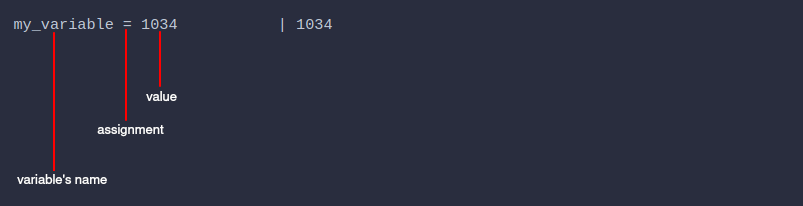# Extended features

We are constantly improving the application, adding more valuable features to it.

### Custom named variables#

When reusable results are not enough, it's handy to use variables.

To declare a variable you need to give it a name and assign a value to that name.In the example below we assign three variables:

my_variable = 1034 | 1034
weight_of_the_box = 25Kg | 25 Kg
weight_of_the_bag = 17Kg | 17 Kg

Further we can use them in our calculations:

my_variable + 15 | 1049
weight = weight_of_the_box + weight_of_the_bag | 42 Kg
weight | 42 Kg

After assigning value to a variable, it can be used in further calculations.

As with reusable results, changing the variable will trigger changes in all dependent calculations.

### Custom named calculations (functions)#

If you have a formula that is frequently repeated across calculations, it is convenient to move it to a function, the so-called custom function.

Custom functions are like macros in excel.

In the example below, we created a BMI function.Here is a detailed usage example: